Next: Exact and Inexact Differentials Up: Heat and Work Previous: Microscopic Interpretation of Heat

# Quasi-Static Processes

Consider the special case of an interaction of the systemwith its surroundings that is carried out so slowly thatremains arbitrarily close to equilibrium at all times. Such a process is said to be quasi-static for the system. In practice, a quasi-static process must be carried out on a timescale that is much longer than the relaxation time of the system. Recall that the relaxation time is the typical timescale for the system to return to equilibrium after being suddenly disturbed. (See Section 3.5.)

A finite quasi-static change can be built up out of many infinitesimal changes. The infinitesimal heat,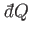, absorbed by the system when infinitesimal work,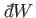, is done on its environment, and its average energy changes by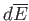, is given by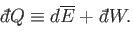(4.11)

The special symbolsandare introduced to emphasize that the work done, and the heat absorbed, are infinitesimal quantities that do not correspond to the difference between two works or two heats. Instead, the work done, and the heat absorbed, depend on the interaction process itself. Thus, it makes no sense to talk about the work in the system before and after the process, or the difference between these.

If the external parameters of the system have the values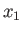,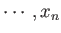then the energy of the system in a definite microstate,, can be written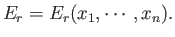(4.12)

Hence, if the external parameters are changed by infinitesimal amounts, so that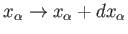for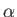in the range 1 to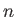, then the corresponding change in the energy of the microstate is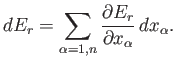(4.13)

The work,, done by the system when it remains in this particular stateis [see Equation (4.8)]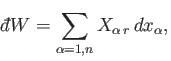(4.14)

where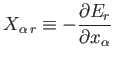(4.15)

is termed the generalized force (conjugate to the external parameter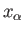) in the state. (See Section B.2.) Note that ifis a displacement then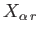is an ordinary force.

Consider, now, an ensemble of systems. Provided that the external parameters of the system are changed quasi-statically, the generalized forceshave well-defined mean values that are calculable from the distribution of systems in the ensemble characteristic of the instantaneous macrostate. The macroscopic work,, resulting from an infinitesimal quasi-static change of the external parameters is obtained by calculating the decrease in the mean energy resulting from the parameter change. Thus,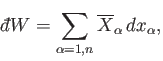(4.16)

where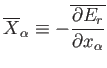(4.17)

is the mean generalized force conjugate to. The mean value is calculated from the equilibrium distribution of systems in the ensemble corresponding to the external parameter values. The macroscopic work,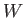, resulting from a finite quasi-static change of external parameters can be obtained by integrating Equation (4.16).

The most well-known example of quasi-static work in thermodynamics is that done by pressure when the volume changes. For simplicity, suppose that the volumeis the only external parameter of any consequence. The work done in changing the volume fromto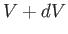is simply the product of the force and the displacement (along the line of action of the force). By definition, the mean equilibrium pressure,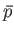, of a given macrostate is equal to the normal force per unit area acting on any surface element. Thus, the normal force acting on a surface element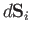is. Suppose that the surface element is subject to a displacement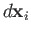. The work done by the element is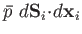. The total work done by the system is obtained by summing over all of the surface elements. Thus,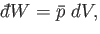(4.18)

where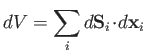(4.19)

is the infinitesimal volume change due to the displacement of the surface. It follows from Equations (4.16) and (4.18) that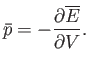(4.20)

Thus, the mean pressure is the generalized force conjugate to the volume,.

Suppose that a quasi-static process is carried out in which the volume is changed from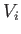to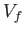. In general, the mean pressure,, is a function of the volume, so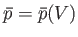. It follows that the macroscopic work done by the system is given by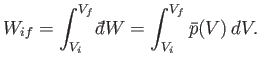(4.21)

This quantity is just the area under the curve in a plot of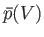versus.Next: Exact and Inexact Differentials Up: Heat and Work Previous: Microscopic Interpretation of Heat
Richard Fitzpatrick 2016-01-25